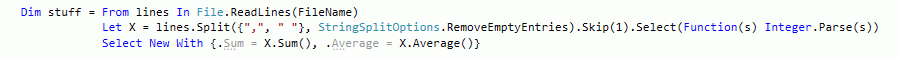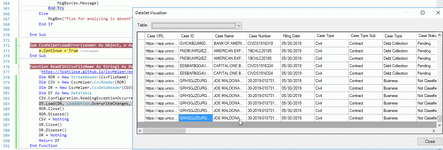﻿ ASP NET, NET Framework, MONO, SQL, Visual Studio | Professional Programming | Viacheslav Eremin
(NET) NET (2018)

## Processing CSV files by CsvHelper.

CSV files is a simple text file, therefore it can be read by any way, for example this LINQ query create IEnumerable interface for CSV files contains rowheader and some digit. By one string of code CSV file has been read and has been calculate average and summary for each line.Because this task is so simple, and CSV files is so spread, there are a lot of library to read CSV LinqToCsv, Linq.Csv, CsvTools. LINQ-to-CSV-library etc, but in this page I show template to use another library CsvHelper.

This library can read CSV to typed memory (class with field definition) and to untyped dynamic memory (DataTable), this is documentation for this library https://joshclose.github.io/CsvHelper/examples.

This is my template to read CSV by this lib to DataTable.` 346:      Function ReadCSV(CsvFileName As String) As DataTable`
` 347:          ErrRowListNumber = New List(Of Integer)`
` 348:          Dim RDR = New StreamReader(CsvFileName)`
` 349:          Dim CSV = New CsvHelper.CsvReader(RDR)`
` 350:          CSV.Configuration.BadDataFound = (Sub(X) MsgBox("Import this row is impossible (incorrect delimiter):" & vbCrLf & X.RawRecord))`
` 351:          CSV.Configuration.Delimiter = ";"`
` 352:          Dim DR = New CsvHelper.CsvDataReader(CSV)`
` 353:          Dim DT As New DataTable()`
` 354:          DT.Load(DR, LoadOption.OverwriteChanges, AddressOf CsvHelperLoadError)`
` 355:          RDR.Close()`
` 356:          RDR.Dispose()`
` 357:          CSV = Nothing`
` 358:          DR.Close()`
` 359:          DR.Dispose()`
` 360:          DR = Nothing`
` 361:          Return DT`
` 362:      End Function`
` 363:   `
` 364:      Dim ErrRowListNumber As List(Of Integer)`
` 365:      Sub CsvHelperLoadError(sender As Object, e As FillErrorEventArgs)`
` 366:          e.DataTable.Rows.Add()`
` 367:          Dim Str1 As New Text.StringBuilder`
` 368:          For i As Integer = 0 To e.Values.Count - 1`
` 369:              Str1.AppendLine("(" & i & ")" & e.Values(i))`
` 370:          Next`
` 371:          MsgBox("Import this row is impossible (more than " & e.Values.Count & " column):" & vbCrLf & Str1.ToString)`
` 372:          ErrRowListNumber.Add(e.DataTable.Rows.Count)`
` 373:          e.Continue = True`
` 374:      End Sub`

Because most problem in practice is malformed CSV, this reader has two callback to process malformed CSV rows - CsvHelperLoadError and expression in string 354. However, I still don't know how to processed malformed row by own code and how to add it to datatable and I'm forced to ignore malformed rows question.

But when data has been read to Datatable it can by filtered and sorted so simple. For example, this is simplest way to use Datatable - only ordered rows.

` 670:          Dim DT1 As DataTable = ReadCSV(IO.Path.Combine(SelectedTempPath, WestlawSelectedListViewFileName))`
` 671:          Dim DV2 As DataView = DT1.DefaultView`
` 672:          DV2.Sort() = e.Argument & " asc"`
` 673:          Dim DT2 As DataTable = DV2.ToTable`
` ....   `

And this is fragment of my real code from another project to do filtered and ordered rows in DataTable.

` 380:                  Dim DT As DataTable = ReadCSV(OutFileName)`
` 381:                  Dim DTF As DataTable = FilterRowAndColumn(DT, UnicourtOrderComboBox.SelectedItem)`
` ....   `
` 427:      Function FilterRowAndColumn(DT As DataTable, SortBy As String) As DataTable`
` 428:          Dim ERC1 As EnumerableRowCollection(Of DataRow) = DT.AsEnumerable.Where(Function(X) X.Field(Of String)("Party Type") <> "Defendant")`
` 429:          Dim DT1 As DataTable = ERC1.CopyToDataTable()`
` 430:          Dim ERC2 As EnumerableRowCollection(Of DataRow) = DT1.AsEnumerable.Where(Function(X) X.Field(Of String)("Party Representation Type") <> "Attorney Represented")`
` 431:          Dim DT2 As DataTable = ERC2.CopyToDataTable()`
` 432:          Dim DT3 As DataTable`
` 433:          Dim ERC3 As EnumerableRowCollection(Of DataRow)`
` 434:          If AllowCompaniesCheckBox.Checked And IncludeIndividualCheckBox.Checked Then`
` 435:              ERC3 = DT2.AsEnumerable.Where(Function(X) X.Field(Of String)("Party Entity Type") = "Company" Or X.Field(Of String)("Party Entity Type") = "Individual")`
` 436:              DT3 = ERC3.CopyToDataTable()`
` 437:          ElseIf AllowCompaniesCheckBox.Checked And Not IncludeIndividualCheckBox.Checked Then`
` 438:              ERC3 = DT2.AsEnumerable.Where(Function(X) X.Field(Of String)("Party Entity Type") = "Company")`
` 439:              DT3 = ERC3.CopyToDataTable()`
` 440:          ElseIf Not AllowCompaniesCheckBox.Checked And IncludeIndividualCheckBox.Checked Then`
` 441:              ERC3 = DT2.AsEnumerable.Where(Function(X) X.Field(Of String)("Party Entity Type") = "Individual")`
` 442:              DT3 = ERC3.CopyToDataTable()`
` 443:          ElseIf Not AllowCompaniesCheckBox.Checked And Not IncludeIndividualCheckBox.Checked Then`
` 444:              DT3 = DT2`
` 445:          End If`
` 446:          'DataTable.Clone function only the schema will be copied. But DataTable.Copy() copies both the structure and data`
` 447:          Dim DT4 As New DataTable`
` 448:          DT4.Columns.Add("Party Name", GetType(String))`
` 449:          DT4.Columns.Add("Source", GetType(String))`
` 450:          DT4.Columns.Add("Street Address", GetType(String))`
` 451:          DT4.Columns.Add("City", GetType(String))`
` 452:          DT4.Columns.Add("State", GetType(String))`
` 453:          DT4.Columns.Add("Zip", GetType(String))`
` 454:          DT4.Columns.Add("URL", GetType(String))`
` 455:          DT4.Columns.Add("ID", GetType(String))`
` 456:          DT4.Columns.Add("Name", GetType(String))`
` 457:          DT4.Columns.Add("Number", GetType(String))`
` ....   `
` 521:          For K As Integer = 0 To DT3.Rows.Count - 1`
` 522:              DT4.Rows.Add()`
` 523:              For I As Integer = 0 To DT4.Columns.Count - 1`
` 524:                  For J As Integer = 0 To DT3.Columns.Count - 1`
` 525:                      If DT4.Columns(I).ColumnName = DT3.Columns(J).ColumnName.Replace("Case ", "") Then`
` 526:                          DT4(DT4.Rows.Count - 1)(I) = DT3(K)(J)`
` 527:                          GoTo NextRow`
` 528:                      End If`
` 529:                  Next`
` 530:  NextRow:`
` 531:              Next`
` 532:          Next`
` 533:          Dim DV4 As DataView = DT4.DefaultView`
` 534:          DV4.Sort() = SortBy & " asc"`
` 535:          Dim DT5 As DataTable = DV4.ToTable`
` 536:          Return DT5`
` 537:      End Function`

<00>  <01>  <02>  <03>  <04>  <05>  <06>  <07>  <08>  <09>  <10>  <11>  <12>  <13>  <14>  <15>  <16>  <17>  <18>  <19